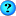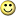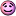WorldViz User Forum(beginner) calculating orientation change with eulers
 Register FAQ Search Today's Posts Mark Forums Read

#1
 astullMember Join Date: Dec 2005 Posts: 9(beginner) calculating orientation change with eulers

I'm capturing Euler angles before and after a user manipulation. The object is fixed in location and only changes in orientation. What I want to measure is the error between a target orientation and the user's response orientation for the object. I've calculated the difference for each of the 3 axis but, if I understand correctly, this doesn't give a measure of the orientation change in 3D space. What is the proper method/strategy to attack this question? I'd like one measure of orientation error but I'm not sure how to get it.#2
 farshizzoWorldViz Team Member Join Date: Mar 2003 Posts: 2,849
Using the euler angles, you can find the look vector of each orientation, then compute the angle difference between these vectors. However, this method will not take roll into account. For example, if both orientations are looking straight ahead, but one has the head rolled 90 degrees, the difference will still be 0 degrees. If you are interested in the roll, then you can also extract the up vector of each orientation and compute the angle difference between these. It would then be up to you to figure out how to convert these two angle differences into some orientation error metric.

Let me know if you are interested in this method and I will supply you with some sample code.
#3
 astullMember Join Date: Dec 2005 Posts: 9Thank you

Yes, this method makes sense and sample code would be welcomed.
#4
 farshizzoWorldViz Team Member Join Date: Mar 2003 Posts: 2,849
Here you goCode:
```import viz
viz.go()

euler1 = [90,0,0]
euler2 = [91,0,5]

def ComputeAngleDifference(euler1, euler2):
m = viz.Transform()

#Extract forward/up vector from euler1
m.setRot( vizmat.EulerToAxisAngle(euler1) )
d = m.get()
forward1 = d[8:11]
up1 = d[4:7]

#Extract forward/up vector from euler2
m.setRot( vizmat.EulerToAxisAngle(euler2) )
d = m.get()
forward2 = d[8:11]
up2 = d[4:7]

#Compute angle difference between forward/up vectors
forward_diff = vizmat.AngleBetweenVector(forward1,forward2)
up_diff = vizmat.AngleBetweenVector(up1,up2)

return forward_diff,up_diff

print ComputeAngleDifference(euler1,euler2)```
#5
 astullMember Join Date: Dec 2005 Posts: 9
non-unique solution

Jerry pointed out that the script doesn't always give a unique solution. For example, both of the following sets of eulers give the same forward_diff and up_diff solution, which is (90, 90).

euler1 [0, 90, 0]
euler2 [0, 0, 0]

and

euler1 [0, 90, 30]
euler2 [0, 0, 0]

Is there a way to address this?
#6
 farshizzoWorldViz Team Member Join Date: Mar 2003 Posts: 2,849
I'm not sure what the problem is. The solution you are getting for those sets of euler angles is correct. The problem with getting non-unique solutions is when extracting euler angles from a matrix. In this example we are creating a matrix from euler angles, so there should be no problem.
#7
 astullMember Join Date: Dec 2005 Posts: 9
3 vectors?

Is the non-unique solution due to the selection of 90 degrees as the pitch angle? Ultimately, I need a unique solution. Is it reasonable to assume that this might be addressed by calculating the difference for a 3rd vector? I don't know the math so I'm only assuming the following modified script would retrieve the necessary values from the matrix to calculate a 3rd vector:

#Extract forward/up vector from euler1
m.setRot( vizmat.EulerToAxisAngle(euler1) )
d = m.get()
forward1 = d[8:11]
up1 = d[4:7]
side1 = d[12:3]

#Extract forward/up vector from euler2
m.setRot( vizmat.EulerToAxisAngle(euler2) )
d = m.get()
forward2 = d[8:11]
up2 = d[4:7]
side2 = d[12:3]

Am I wondering in the dark?Alternatively, wouldn't a unique solution also result if I calculated the displacement from the facing direction AND the angle of rotation about that axis (the facing direction)?
#8
 farshizzoWorldViz Team Member Join Date: Mar 2003 Posts: 2,849
Yes, you could also compute the angle difference between the side vectors as well. Here is a modified version of the sample code that also returns the difference between the side vectors:
Code:
```import viz
viz.go()

euler1 = [0,0,0]
euler2 = [0,90,30]

def ComputeAngleDifference(euler1, euler2):
m = viz.Transform()

#Extract forward/up/side vector from euler1
m.setRot( vizmat.EulerToAxisAngle(euler1) )
d = m.get()
forward1 = d[8:11]
up1 = d[4:7]
side1 = d[:3]

#Extract forward/up/side vector from euler2
m.setRot( vizmat.EulerToAxisAngle(euler2) )
d = m.get()
forward2 = d[8:11]
up2 = d[4:7]
side2 = d[:3]

#Compute angle difference between forward/up/side vectors
forward_diff = vizmat.AngleBetweenVector(forward1,forward2)
up_diff = vizmat.AngleBetweenVector(up1,up2)
side_diff = vizmat.AngleBetweenVector(side1,side2)

return forward_diff,up_diff,side_diff

print ComputeAngleDifference(euler1,euler2)```
Quote:
 Alternatively, wouldn't a unique solution also result if I calculated the displacement from the facing direction AND the angle of rotation about that axis (the facing direction)?
Yes, this should provide a unique solution as well. I'll write up a sample script that does this and post it shortly.
#9
 astullMember Join Date: Dec 2005 Posts: 9
another hole

I don't mean to be a bother but the modified script still yields a non-unique solution. For example, both of the following sets of eulers yield the same solution:

euler1 = [30,90,0]
euler2 = [0,0,0]

euler1 = [0,90,30]
euler2 = [0,0,0]

solution: (90, 90, 30)

I don't understand why this happens. Maybe the vector/rotation alternative may be a more practical solution.

Thank you again for your help!
#10
 farshizzoWorldViz Team Member Join Date: Mar 2003 Posts: 2,849
Those values are correct. I'm not sure what you are expecting. There is always going to be multiple euler sets that will yield the same angle difference. The vector/rotation alternative will also yield the same value for multiple sets. For example, the vector/rotation difference between the following sets will be the same:
Code:
```euler1 = [0,0,0]
euler2 = [90,0,0]

#Vector difference will be 90
#Rotation difference will be 0

euler1 = [90,0,0]
euler2 = [180,0,0]

#Vector difference will be 90
#Rotation difference will be 0```
From your first post it seems you are interested in the relative error between the two orientations. I don't see how having non-unique solutions affects this. Maybe I'm misunderstanding your original request.
#11
 astullMember Join Date: Dec 2005 Posts: 9
Yes

Yes, indeed! There are likely to be multiple euler sets that yield the same displacement errors. Sorry for being so bone-headed.#12
 farshizzoWorldViz Team Member Join Date: Mar 2003 Posts: 2,849
No worries. I know how confusing all this 3d math can get.

Would you still like me to provide you with the vector/rotation alternative?
#13
 astullMember Join Date: Dec 2005 Posts: 9

Yes, it would be helpful if you could offer a script for calculating the vector/rotation. Fewer variables makes the analysis much easier.

Thank you.

Last edited by astull; 02-16-2007 at 04:54 PM.
#14
 farshizzoWorldViz Team Member Join Date: Mar 2003 Posts: 2,849
Here is the vector/rotation code:
Code:
```import viz
viz.go()

euler1 = [0,0,30]
euler2 = [0,5,-30]

def ComputeAngleDifference(euler1, euler2):
m = viz.Transform()

#Extract forward/up/side vector from euler1
m.setRot( vizmat.EulerToAxisAngle(euler1) )
d = m.get()
forward1 = d[8:11]
up1 = vizmat.Vector(d[4:7])

#Extract up vector from euler1 without roll
m.setRot( vizmat.EulerToAxisAngle(euler1,euler1,0.0) )
d = m.get()
up1_no_roll = vizmat.Vector(d[4:7])

#Compute rotation around forward vector of euler1
roll1 = vizmat.AngleBetweenVector(up1,up1_no_roll)
if (up1_no_roll.cross(up1) * forward1) < 0.0:
roll1 *= -1.0

#Extract forward/up/side vector from euler2
m.setRot( vizmat.EulerToAxisAngle(euler2) )
d = m.get()
forward2 = d[8:11]
up2 = vizmat.Vector(d[4:7])

#Extract up vector from euler2 without roll
m.setRot( vizmat.EulerToAxisAngle(euler2,euler2,0.0) )
d = m.get()
up2_no_roll = vizmat.Vector(d[4:7])

#Compute rotation around forward vector of euler2
roll2 = vizmat.AngleBetweenVector(up2,up2_no_roll)
if (up2_no_roll.cross(up2) * forward2) < 0.0:
roll2 *= -1.0

#Compute angle difference between forward/up/side vectors
forward_diff = vizmat.AngleBetweenVector(forward1,forward2)
rotation_diff = abs(vizmat.AngleDiff(roll1,roll2))

return forward_diff,rotation_diff

print ComputeAngleDifference(euler1,euler2)```
#15
 astullMember Join Date: Dec 2005 Posts: 9
quatdiff c code?

I met with Andy B. today and he pointed me to the quatdiff function as a potential single metric solution to the error calculation. I know the function is available through Vizard but I'd like to verify it on paper. He said that you might be able to send me the C code.

Thanks again!
#16
 farshizzoWorldViz Team Member Join Date: Mar 2003 Posts: 2,849
The QuatDiff function simply uses the above method of computing the angle difference between the forward and up vectors, and returns the maximum of those two values.

You can modify the sample code I gave you to replicate this behavior.
#17
 farshizzoWorldViz Team Member Join Date: Mar 2003 Posts: 2,849
Here is some code that calculates the difference between two angles based on the method steve described:
Code:
```import viz
import math
viz.go()

euler1 = [90,0,0]
euler2 = [0,0,90]

def ComputeAngleDifference(euler1, euler2):
q1 = viz.Quat( vizmat.EulerToQuat(euler1) )
q2 = viz.Quat( vizmat.EulerToQuat(euler2) )

delta = q1 * q2.inverse()

return viz.degrees( math.acos( delta.w ) ) * 2.0

print ComputeAngleDifference(euler1,euler2)```
I'll updated Vizard's QuatDiff function to use this method in the next release.

 Thread ToolsShow Printable VersionEmail this Page Display Modes Rate This ThreadLinear ModeSwitch to Hybrid ModeSwitch to Threaded Mode Rate This Thread: 5 : Excellent 4 : Good 3 : Average 2 : Bad 1 : TerriblePosting Rules You may not post new threads You may not post replies You may not post attachments You may not edit your posts BB code is On Smilies are On [IMG] code is On HTML code is Off Forum Rules
 Forum Jump User Control Panel Private Messages Subscriptions Who's Online Search Forums Forums Home Announcements Vizard Precision Position Tracker (PPT) Plug-in development

All times are GMT -7. The time now is 12:53 PM.Copyright 2002-2018 WorldViz LLC